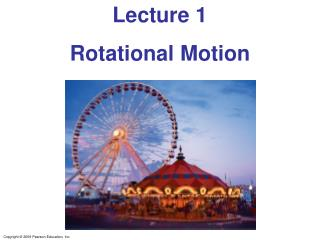Download PresentationLecture 1 Rotational Motion

Loading in 2 Seconds...

# Lecture 1 Rotational Motion - PowerPoint PPT Presentation

Lecture 1 Rotational Motion. Angular Quantities Vector Nature of Angular Quantities Constant Angular Acceleration Solving Problems in Rotational Dynamics. 10-1 Angular Quantities.I am the owner, or an agent authorized to act on behalf of the owner, of the copyrighted work described.
Download Presentation## Lecture 1 Rotational Motion

An Image/Link below is provided (as is) to download presentation

Download Policy: Content on the Website is provided to you AS IS for your information and personal use and may not be sold / licensed / shared on other websites without getting consent from its author.While downloading, if for some reason you are not able to download a presentation, the publisher may have deleted the file from their server.

- - - - - - - - - - - - - - - - - - - - - - - - - - E N D - - - - - - - - - - - - - - - - - - - - - - - - - -
Presentation Transcript
1. Lecture 1 Rotational Motion

2. Angular Quantities • Vector Nature of Angular Quantities • Constant Angular Acceleration • Solving Problems in Rotational Dynamics

3. 10-1 Angular Quantities In purely rotational motion, all points on the object move in circles around the axis of rotation (“O”). The radius of the circle is R. All points on a straight line drawn through the axis move through the same angle in the same time. The angle θ in radians is defined: where l is the arc length.

4. 10-1 Angular Quantities Angular displacement: The average angular velocity is defined as the total angular displacement divided by time: The instantaneous angular velocity:

5. 10-1 Angular Quantities The angular acceleration is the rate at which the angular velocity changes with time: The instantaneous acceleration:

6. 10-1 Angular Quantities Every point on a rotating body has an angular velocityω and a linear velocityv. They are related:

7. 10-1 Angular Quantities Conceptual Example 10-2: Is the lion faster than the horse? On a rotating carousel or merry-go-round, one child sits on a horse near the outer edge and another child sits on a lion halfway out from the center. (a) Which child has the greater linear velocity? (b) Which child has the greater angular velocity?

8. 10-1 Angular Quantities Objects farther from the axis of rotation will move faster.

9. 10-1 Angular Quantities If the angular velocity of a rotating object changes, it has a tangential acceleration: Even if the angular velocity is constant, each point on the object has a centripetal acceleration:

10. 10-1 Angular Quantities Here is the correspondence between linear and rotational quantities:

11. 10-1 Angular Quantities The frequency is the number of complete revolutions per second: Frequencies are measured in hertz: The period is the time one revolution takes:

12. 10-1 Angular Quantities Example 10-4: Hard drive. The platter of the hard drive of a computer rotates at 7200 rpm (rpm = revolutions per minute = rev/min). (a) What is the angular velocity (rad/s) of the platter? (b) If the reading head of the drive is located 3.00 cm from the rotation axis, what is the linear speed of the point on the platter just below it? (c) If a single bit requires 0.50 μm of length along the direction of motion, how many bits per second can the writing head write when it is 3.00 cm from the axis?

13. 10-1 Angular Quantities Example 10-5: Given ω as function of time. A disk of radius R = 3.0 m rotates at an angular velocity ω = (1.6 + 1.2t) rad/s, where t is in seconds. At the instant t = 2.0 s, determine (a) the angular acceleration, and (b) the speed v and the components of the acceleration a of a point on the edge of the disk.

14. 10-2 Vector Nature of Angular Quantities The angular velocity vector points along the axis of rotation, with the direction given by the right-hand rule. If the direction of the rotation axis does not change, the angular acceleration vector points along it as well.

15. 10-3 Constant Angular Acceleration The equations of motion for constant angular acceleration are the same as those for linear motion, with the substitution of the angular quantities for the linear ones.

16. 10-3 Constant Angular Acceleration Example 10-6: Centrifuge acceleration. A centrifuge rotor is accelerated from rest to 20,000 rpm in 30 s. (a) What is its average angular acceleration? (b) Through how many revolutions has the centrifuge rotor turned during its acceleration period, assuming constant angular acceleration?

17. Summary of Chapter 10 • Angles are measured in radians; a whole circle is 2π radians. • Angular velocity is the rate of change of angular position. • Angular acceleration is the rate of change of angular velocity. • The angular velocity and acceleration can be related to the linear velocity and acceleration. • The frequency is the number of full revolutions per second; the period is the inverse of the frequency.

18. Summary of Chapter 10, cont. • The equations for rotational motion with constant angular acceleration have the same form as those for linear motion with constant acceleration.